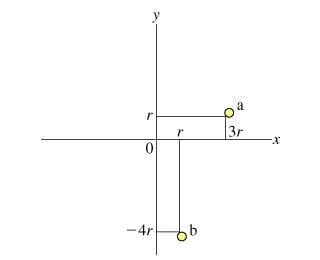# Total Kinetic Energy in a System

• Todd88

## Homework Statement

Using the total moment of inertia of the system, find the total kinetic energy of the system.

Here is the diagram:## Homework Equations

Find the total kinetic energy of the system using m, r, w.

## The Attempt at a Solution

For particle a, I found the x and y components of inertia to be mr^2 and 9mr^2 respectively. I found the total y component of inertia of the system to be 11mr^2. I can't seem to find the total kinetic energy. I thought that if you take the original equation:

K = 1/2mv^2 and plug in the components => K = 1/2m(r^2+9r^2) = 5mr^2 for particle A

K = 1/2mv^2 and plug in the components => K = 1/2m(r^2+16r^2) = 17/2mr^2 for particle B

Then add the two quantities to get 27/2mr^2w^2 for the kinetic energy. This isn't right...anything to help me would be much appreciated! Thanks!